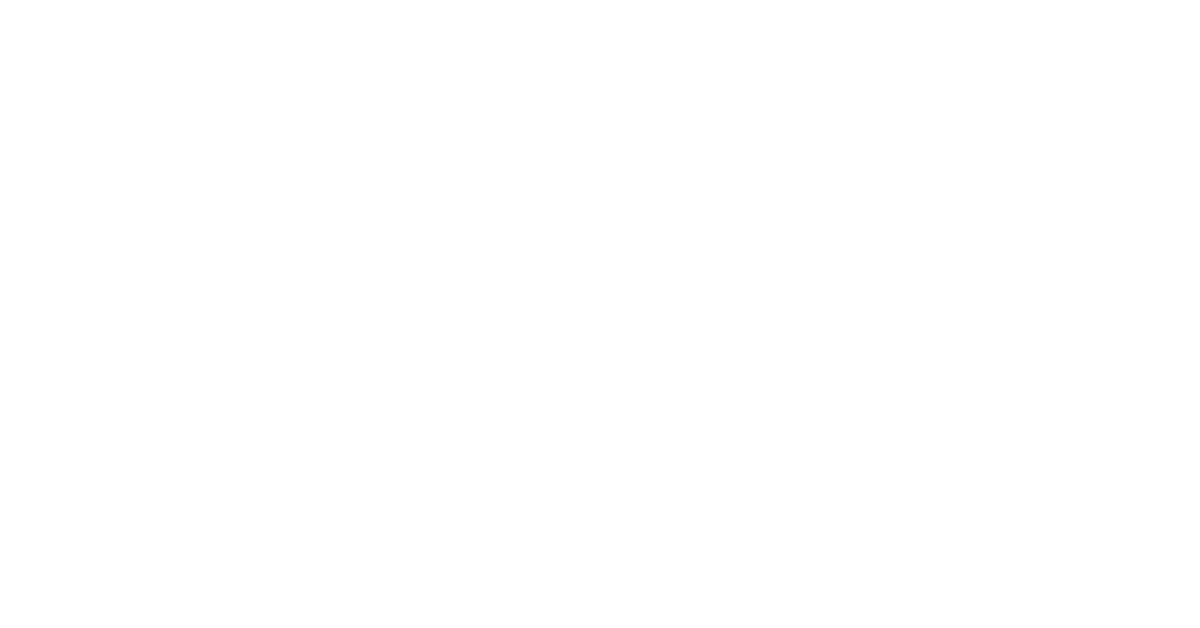# Leaf Disease detection using image processing OpenCV Python

Leaf disease detection using image processing, OpenCV, and Python is a non-invasive and efficient way to detect the diseases in the plant.

There are some benefits for this First, it is much faster, allowing farmers to inspect large fields of crops quickly and easily. Second, it is more accurate, as algorithms can be trained to identify diseases that are difficult to spot with the naked eye. Third, it is non-invasive, meaning that leaves do not need to be damaged or destroyed for testing.

We are going to present the code which use the color segmentation technique to segment the real time frames from the camera. Once frame is captured, it is passed to the color segmenation based code. That code detect the dominant color of the image. Then using a simple rule based approach the disease is predicted with healthy or diseased plant.

## Python Code for Leaf Disease Detection using Color Segmentation

Here is the complete code for this project

```.wp-block-code {
border: 0;
}

.wp-block-code > span {
display: block;
overflow: auto;
}

.shcb-language {
border: 0;
clip: rect(1px, 1px, 1px, 1px);
-webkit-clip-path: inset(50%);
clip-path: inset(50%);
height: 1px;
margin: -1px;
overflow: hidden;
position: absolute;
width: 1px;
word-wrap: normal;
word-break: normal;
}

.hljs {
box-sizing: border-box;
}

.hljs.shcb-code-table {
display: table;
width: 100%;
}

.hljs.shcb-code-table > .shcb-loc {
color: inherit;
display: table-row;
width: 100%;
}

.hljs.shcb-code-table .shcb-loc > span {
display: table-cell;
}

.wp-block-code code.hljs:not(.shcb-wrap-lines) {
white-space: pre;
}

.wp-block-code code.hljs.shcb-wrap-lines {
white-space: pre-wrap;
}

.hljs.shcb-line-numbers {
border-spacing: 0;
counter-reset: line;
}

.hljs.shcb-line-numbers > .shcb-loc {
counter-increment: line;
}

.hljs.shcb-line-numbers .shcb-loc > span {
}

.hljs.shcb-line-numbers .shcb-loc::before {
border-right: 1px solid #ddd;
content: counter(line);
display: table-cell;
text-align: right;
-webkit-user-select: none;
-moz-user-select: none;
-ms-user-select: none;
user-select: none;
white-space: nowrap;
width: 1%;
}
```import cv2
import numpy as np

cap = cv2.VideoCapture(0)
cap.set(3,320)
cap.set(4,240)

def dominateColor(images):
#Check the distribution of red values
v = []
c = ['Red','Green','Blue']
red_value = np.mean(images[:, :, 2])
v.append(red_value)

# Check the distribution of green values

green_value = np.mean(images[:, :, 1])
v.append(green_value)

# Check the distribution of blue values

blue_value = np.mean(images[:, :, 0])
v.append(blue_value)
#print(c[v.index(max(v))])
return v.index(max(v))

def detect_leaf(img):
kernel = np.ones((7,7),np.uint8)
hsv = cv2.cvtColor(img, cv2.COLOR_BGR2HSV)
# find the brown color
mask_brown = cv2.inRange(hsv, (8, 60, 20), (30, 255, 200))
# find the yellow and green color in the leaf
mask_yellow_green = cv2.inRange(hsv, (10, 39, 64), (86, 255, 255))
# find any of the three colors(green or brown or yellow) in the image

redCount = 0
greenCount = 0

while(True):
# Capture frame-by-frame

if ret:
contours, hierarchy = cv2.findContours(mask.copy(), cv2.RETR_EXTERNAL, cv2.CHAIN_APPROX_SIMPLE)
output = frame
if contours:
# find the biggest countour (c) by the area
c = max(contours, key = cv2.contourArea)
x,y,w,h = cv2.boundingRect(c)
crop_img = frame[y:y+h, x:x+w]

#cv2.imshow('new',crop_img)
dc = dominateColor(crop_img)
if dc == 0:
redCount = redCount+1
greenCount = 0
cv2.rectangle(output,(x,y),(x+w,y+h),(0,0,255),2)
if dc == 1:
#hist2 = cv2.calcHist([crop_img],None,,[0,256])
hist2 = cv2.calcHist([crop_img],,None,,[0,256])
histogram, bin_edges = np.histogram(
crop_img[:, :, 1], bins=10, range=(0, 10)
)
if sum(histogram)>140:
greenCount = greenCount+1
redCount = 0
cv2.rectangle(output,(x,y),(x+w,y+h),(0,255,0),2)
else:
redCount = redCount + 1
greenCount = 0
cv2.rectangle(output,(x,y),(x+w,y+h),(0,0,255),2)

#print(histogram)
#hsv_croped = cv2.cvtColor(crop_img, cv2.COLOR_BGR2HSV)
#lower = np.array([22, 93, 0])
#upper = np.array([45, 255, 255])
#cv2.rectangle(output,(x,y),(x+w,y+h),(0,255,0),2)
#cv2.imshow('cim',o2)
if redCount> 10:
redCount = 0
greenCount = 0
print('Deseased Leaf')
elif greenCount>10:
greenCount = 0
redCount = 0
print('Healthy Leaf')
cv2.imshow('frame',output)
if cv2.waitKey(1) & 0xFF == ord('q'):
break
else:
print('no frame')
break;

# When everything done, release the capture
cap.release()
cv2.destroyAllWindows()

```Code language: Python (python)```

This site uses Akismet to reduce spam. Learn how your comment data is processed.

Automated page speed optimizations for fast site performance